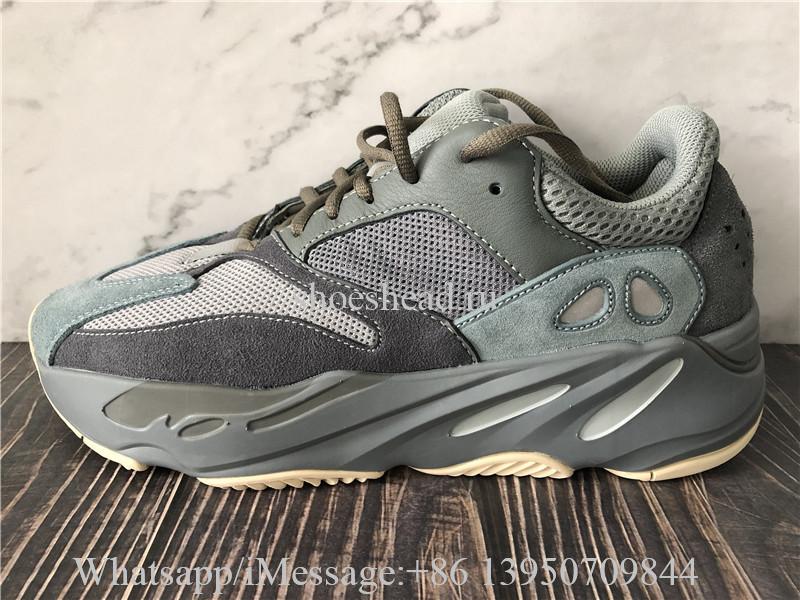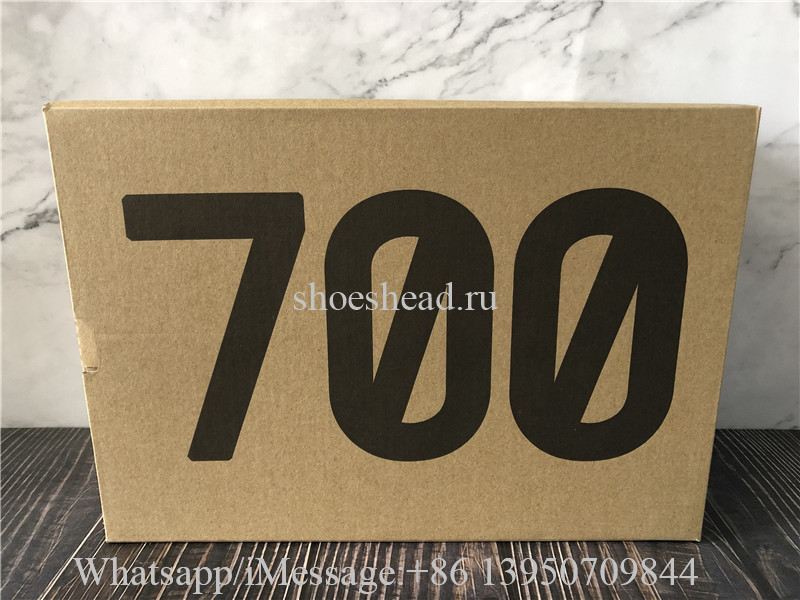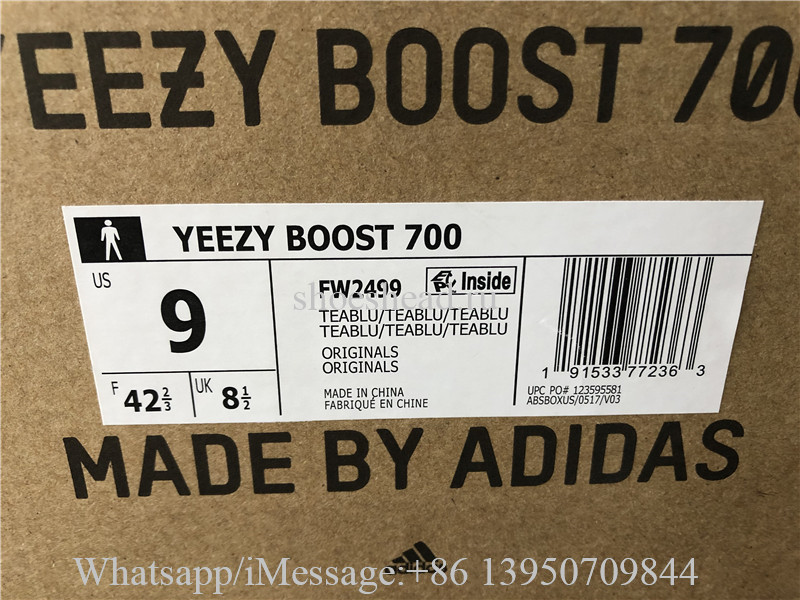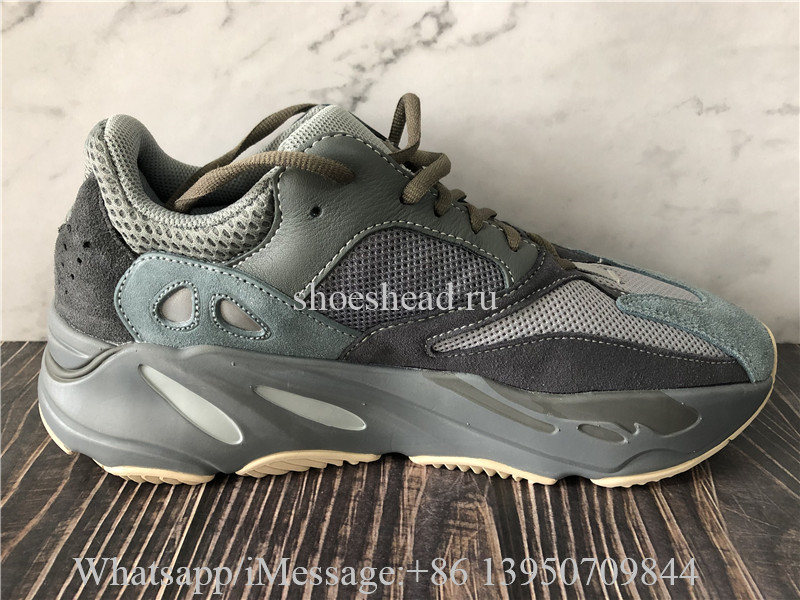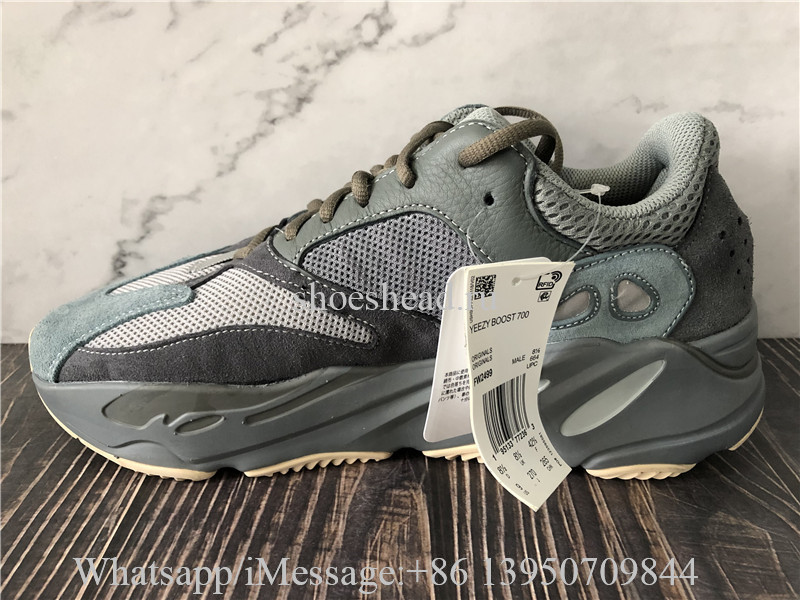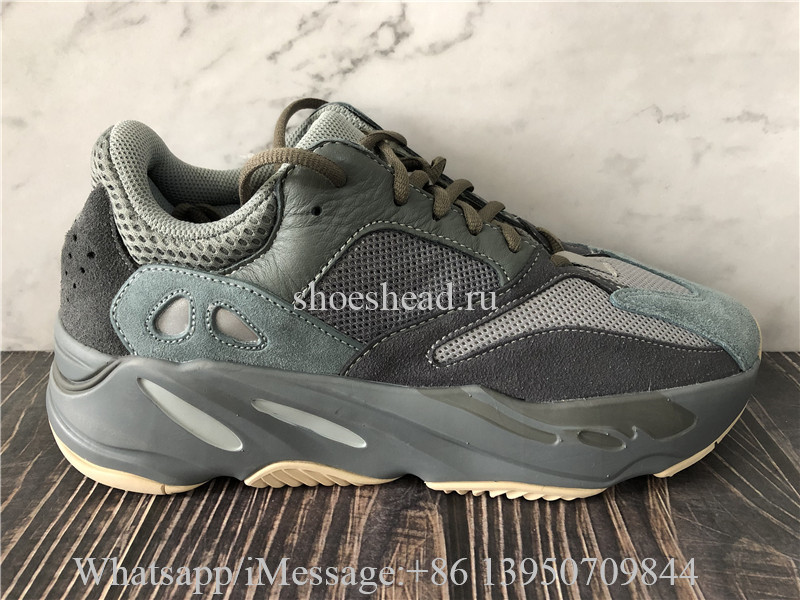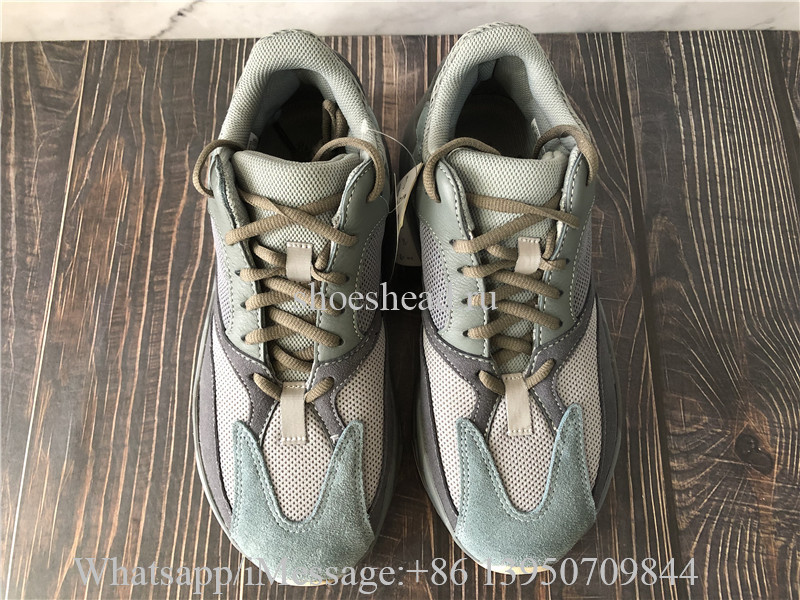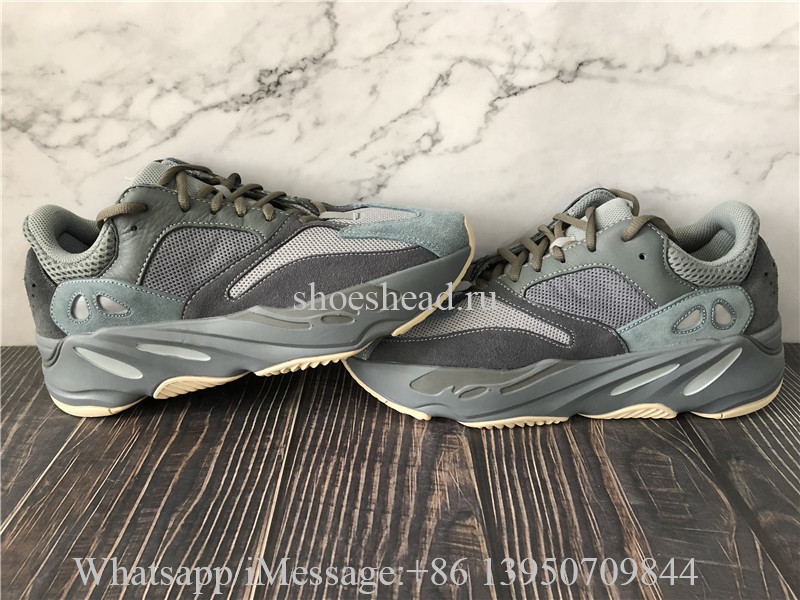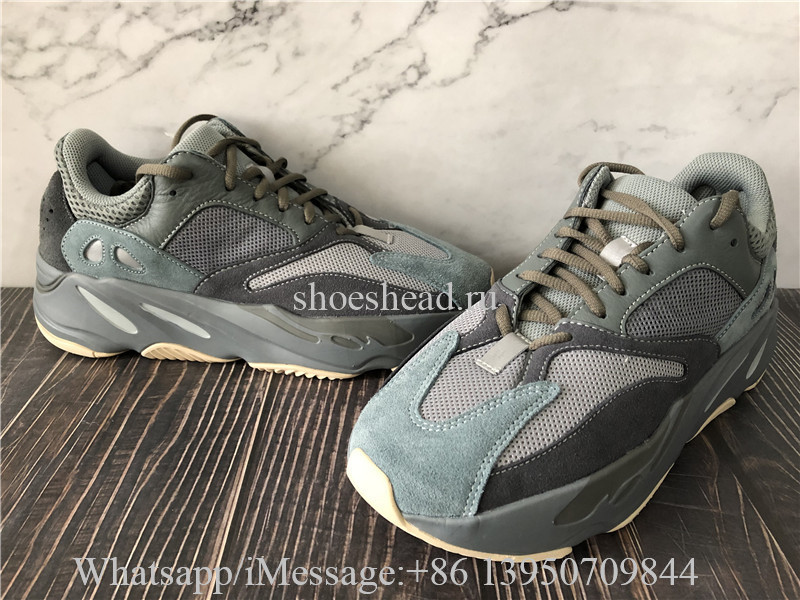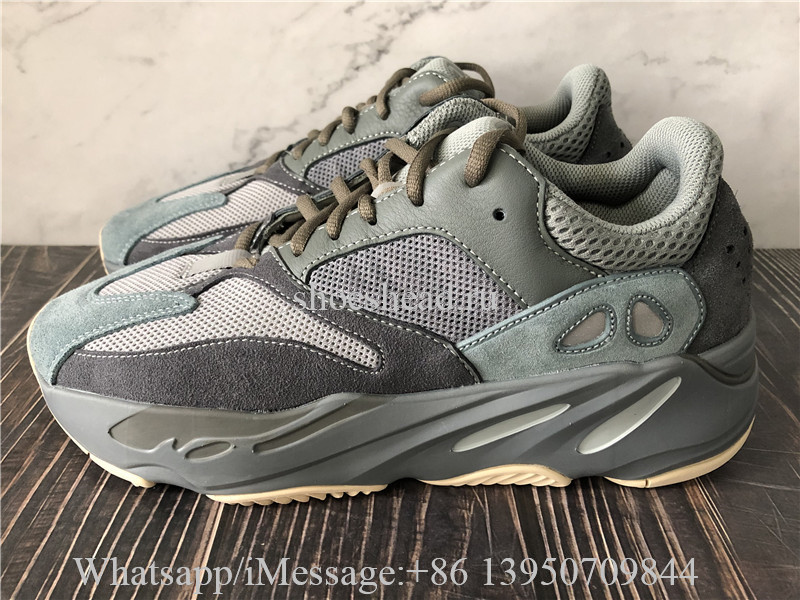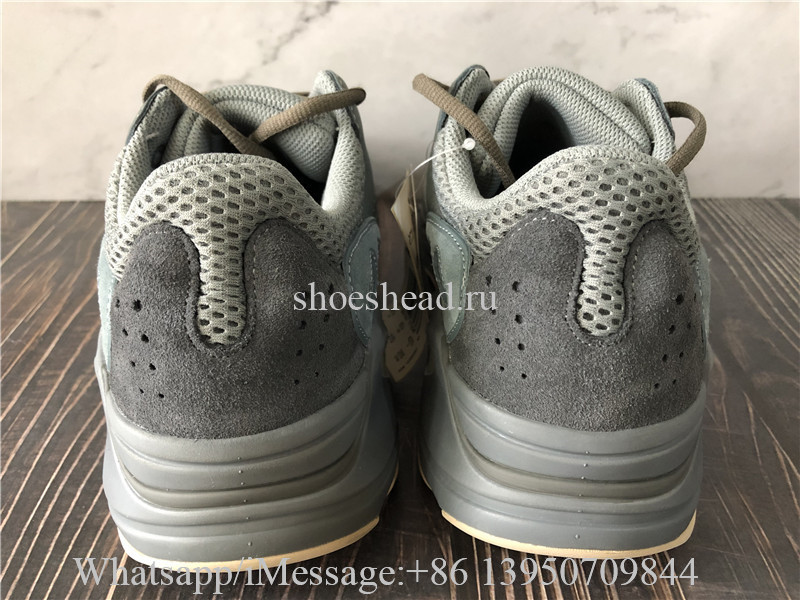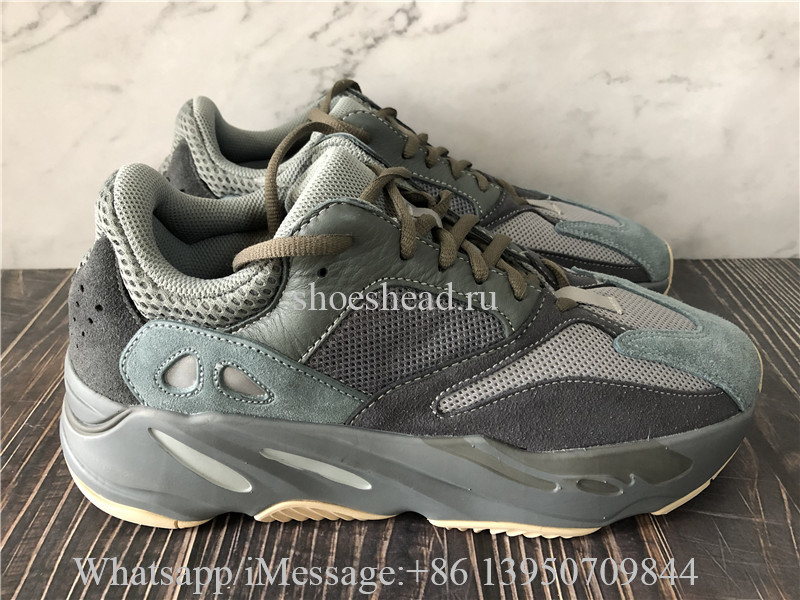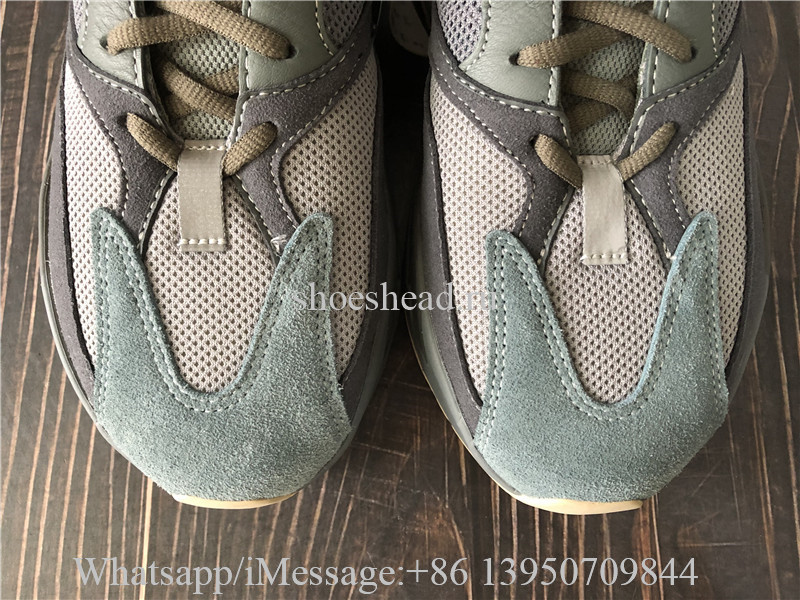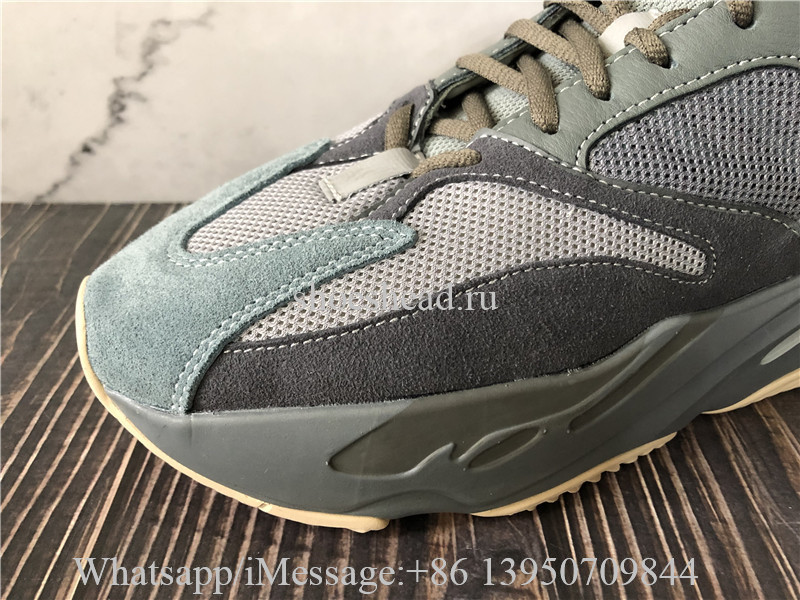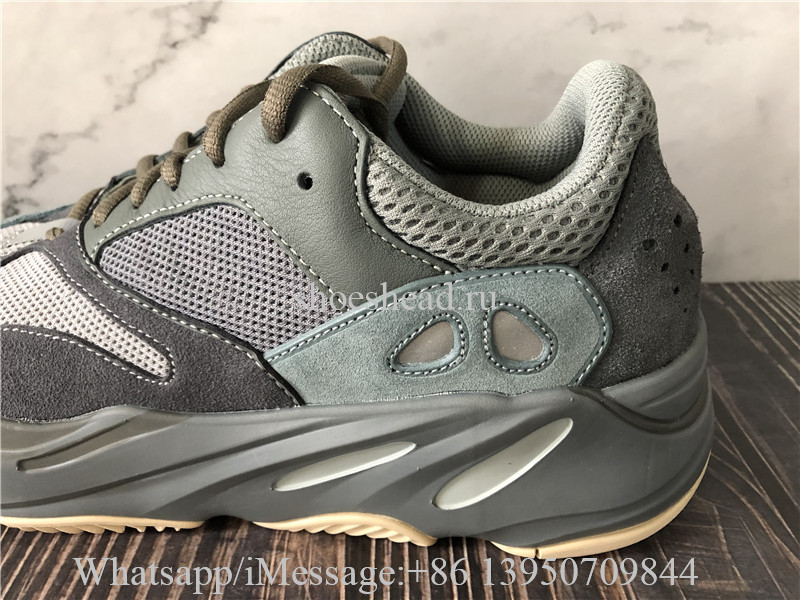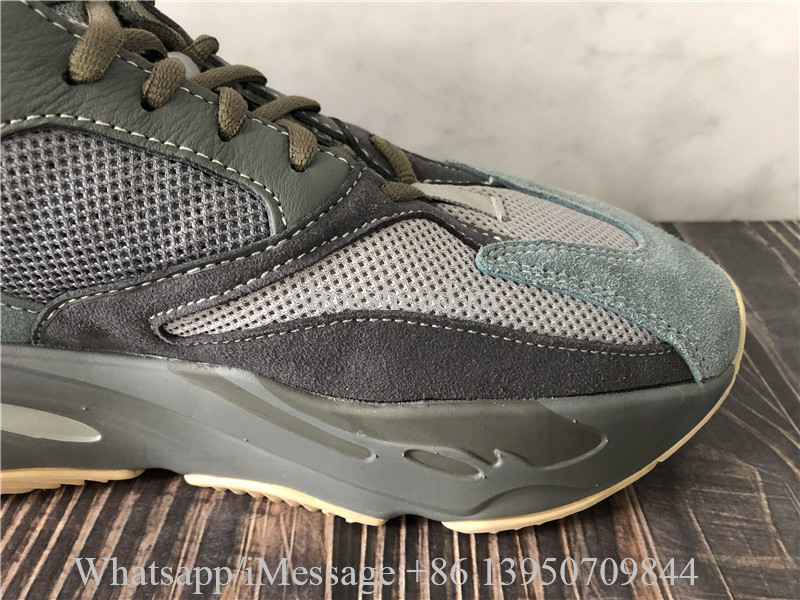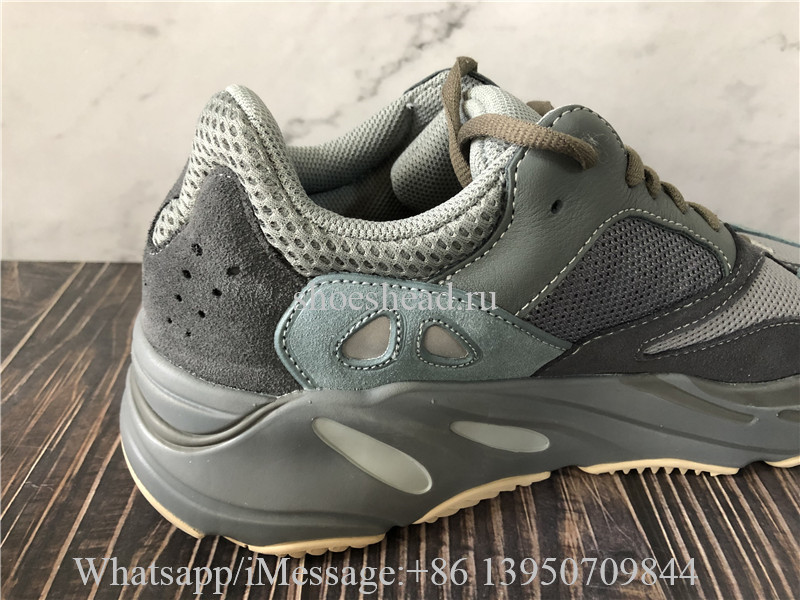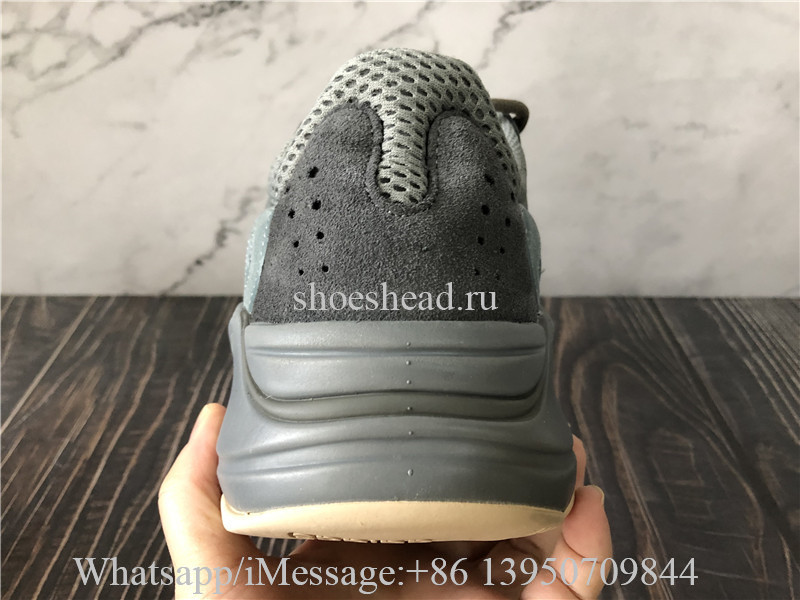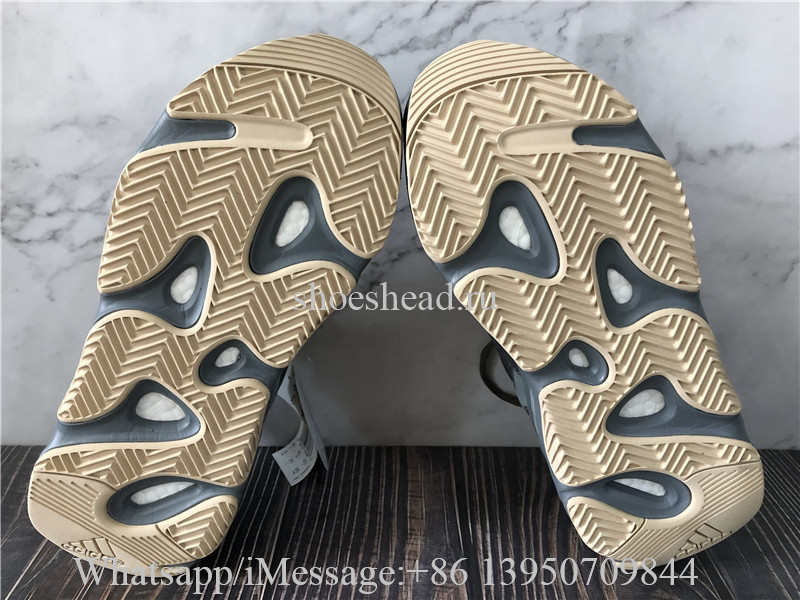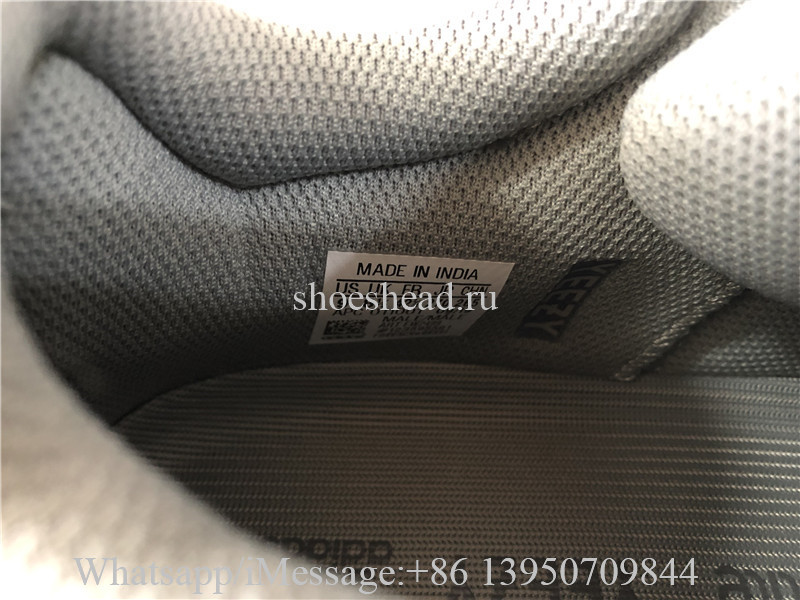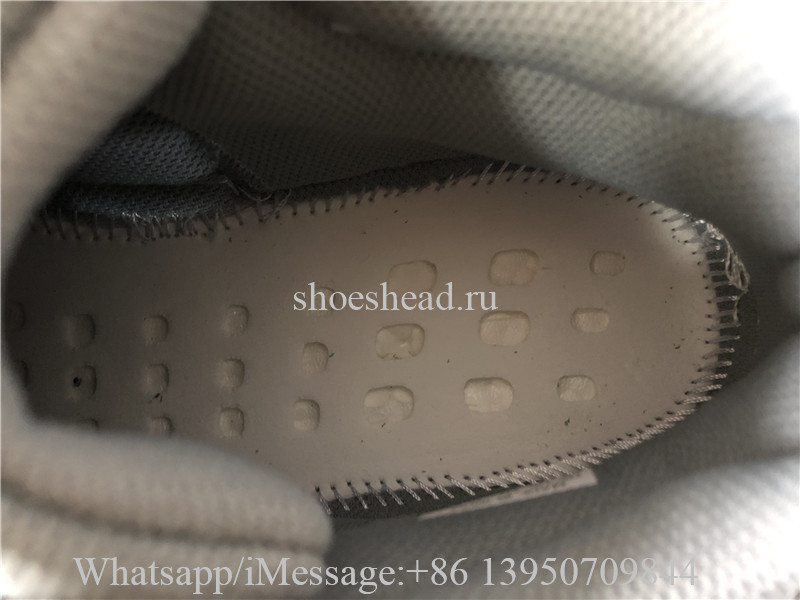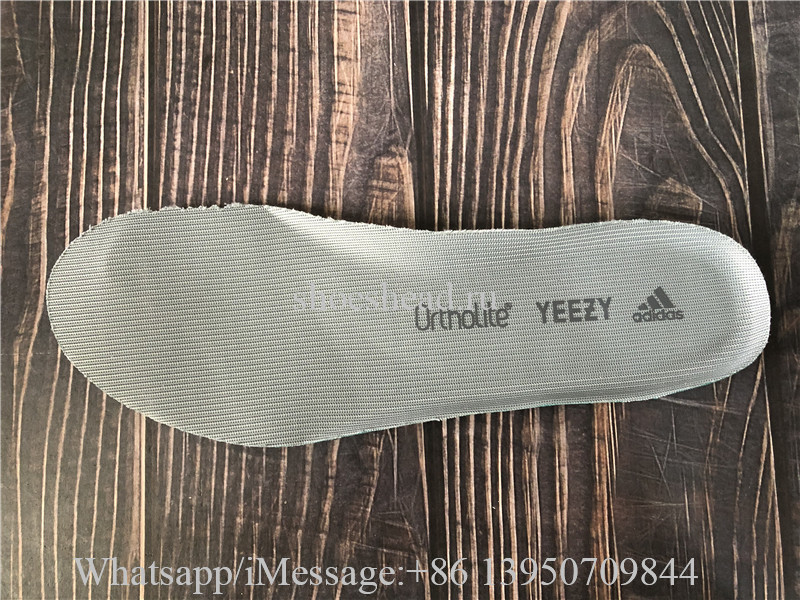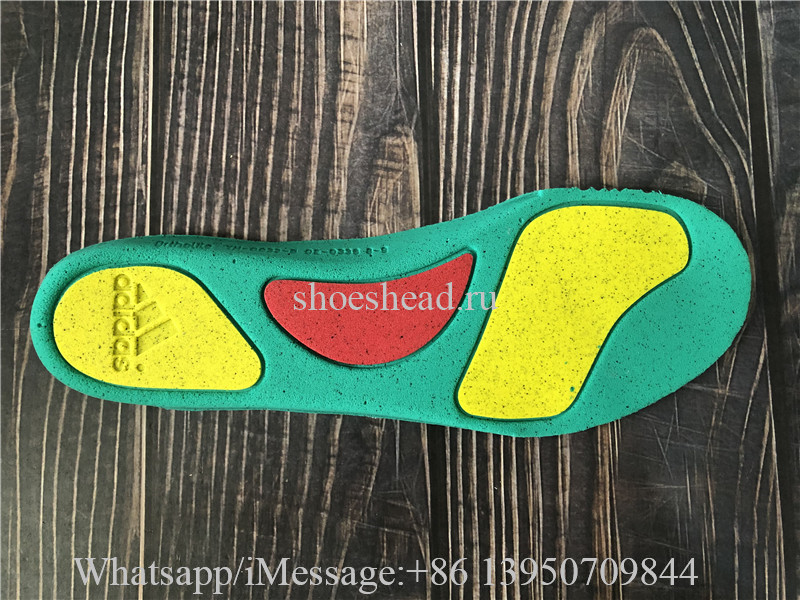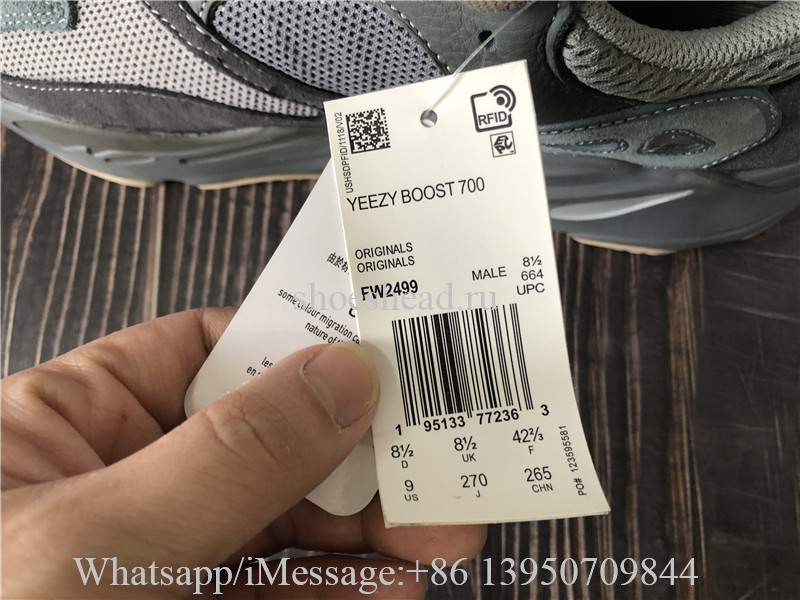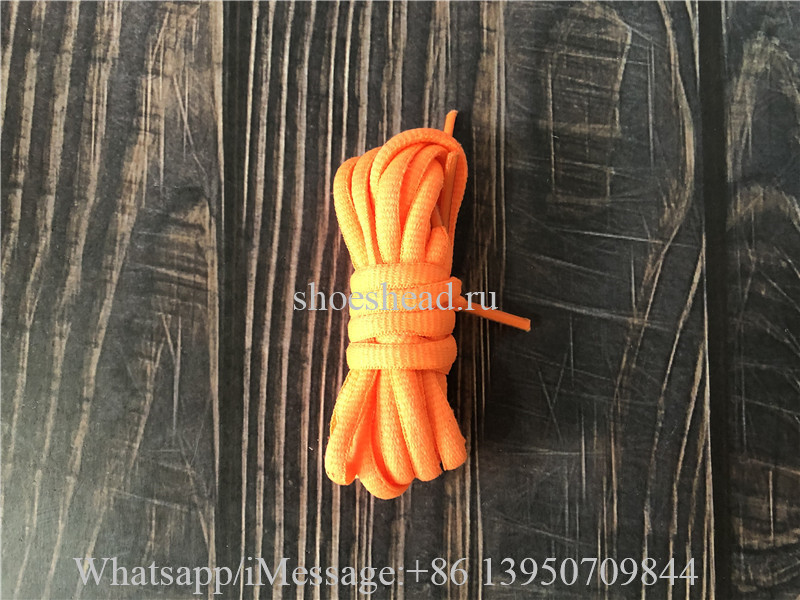Have a nice shopping time on shoeshead.ru
USD
Home>Yeezy>Yeezy Boost 700>Adidas Yeezy Boost 700 Teal Blue FW2499

# Adidas Yeezy Boost 700 Teal Blue FW2499 Item NO.: Yeezy219

Write a Review
US\$ 145.00
women size US4=UK2.5=FR34 2/3=JP210 women size US4.5=UK3=FR35 1/3=JP215 women size US5=UK3.5=FR36=JP220 women size US5.5=UK4=FR36 2/3=JP225 women size US6=UK4.5=FR37 1/3=JP230 women size US6.5=UK5=FR38=JP235 women size US7=UK5.5=FR38 2/3=JP240 women size US7.5=UK6=FR39 1/3=JP245 women size US8=UK6.5=FR40=JP250 women size US8.5=UK7=FR40 2/3=JP255 women size US9=UK7.5=FR41 1/3=JP260 men size US6=UK5.5=FR38 2/3=JP240 men size US6.5=UK6=FR39 1/3=JP245 men size US7=UK6.5=FR40=JP250 men size US7.5=UK7=FR40 2/3=JP255 men size US8=UK7.5=FR41 1/3=JP260 men size US8.5=UK8=FR42=JP265 men size US9=UK8.5=FR42 2/3=JP270 men size US9.5=UK9=FR43 1/3=JP275 men size US10=UK9.5=FR44=JP280 men size US10.5=UK10=FR44 2/3=JP285 men size US11=UK10.5=FR45 1/3=JP290 men size US11.5=UK11=FR46=JP295 men size US12.5=UK12=FR47 1/3=JP305 men size US12=UK11.5=FR46 2/3=JP300 men size US13=UK12.5=FR48=JP310
Quantity